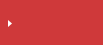>  What is area roughness?  >  Area Roughness Parameters

# Area Roughness Parameters

## ISO 25178 Surface Texture Parameters Explained

• Height
• Spatial
• Hybrid
• Functional
• Functional volume
• FeatureSearch from the parameters list

## Sa (arithmetical mean height)

This parameter is the extension of Ra (arithmetical mean height of a line) to a surface. It expresses, as an absolute value, the difference in height of each point compared to the arithmetical mean of the surface. This parameter is used generally to evaluate surface roughness.## Search from the parameters list

Height Sa (arithmetical mean height) Sz (Maximum height) Sq (Root mean square height) Ssk (Skewness) Sku (Kurtosis) Sp (Maximum peak height) Sv (Maximum pit height) Sal (Auto-correlation length) / Str (Texture aspect ratio) Std* (Texture direction) Sdq (Root mean square gradient) Sdr (Developed interfacial area ratio) Smr(c) Areal material (bearing area) ratio Smc(mr) Inverse areal material ratio Sk (Core roughness depth) Spk (Reduced peak height) Svk (reduced dale height) Smr (Peak material portion) Smr2 (Valley material portion (percentage of the measurement area that comprises the deeper valley structures associated with Svk)) Sxp (Peak extreme height) Vvv (Dale void volume) Vvc (Core void volume) Vmp (Peak material volume) Vmc (Core material volume) Spd (Density of peaks) Spc (Arithmetic mean peak curvature) S10z / S5p / S5v / Sda(c) / Sha(c) / Sdv(c) / Shv(c)